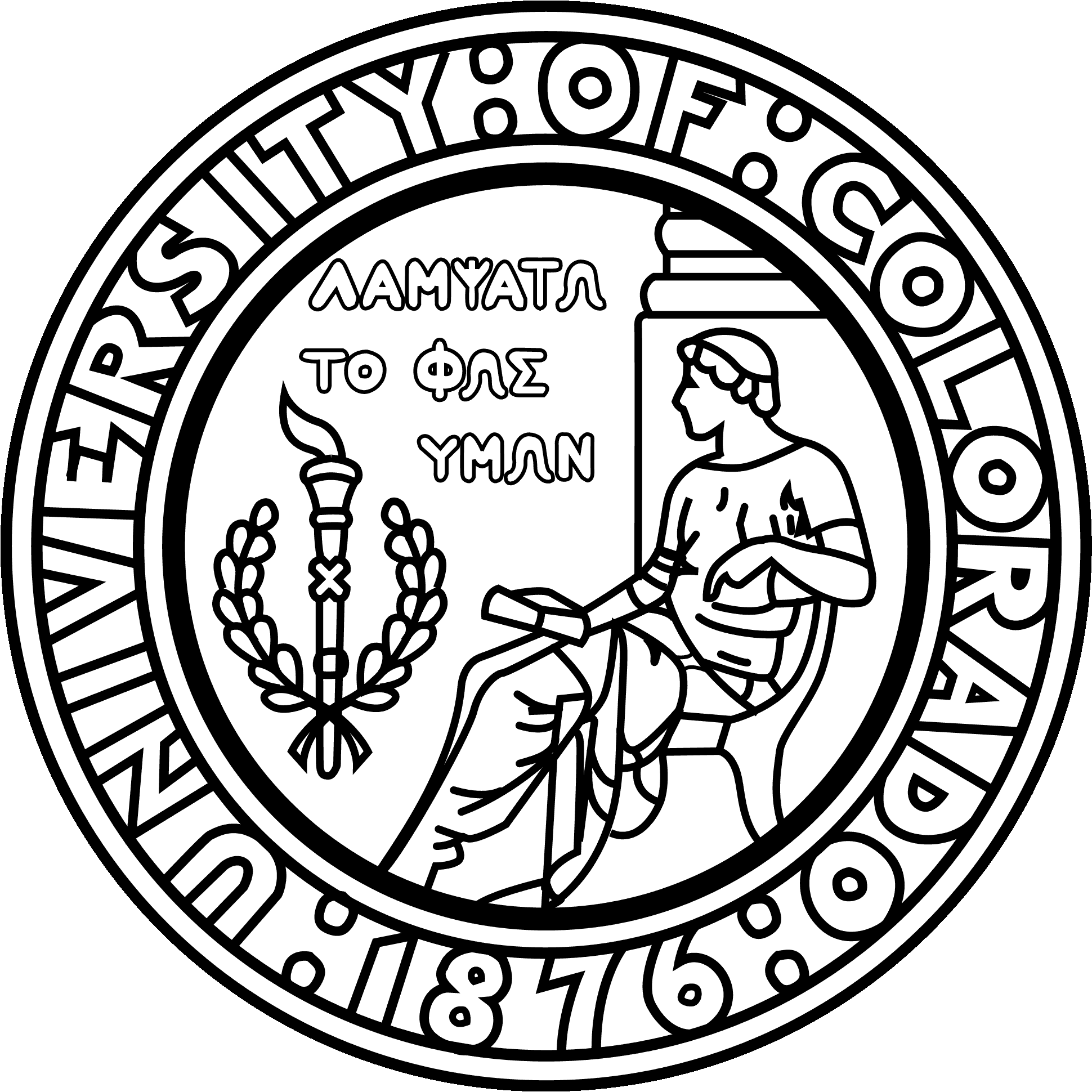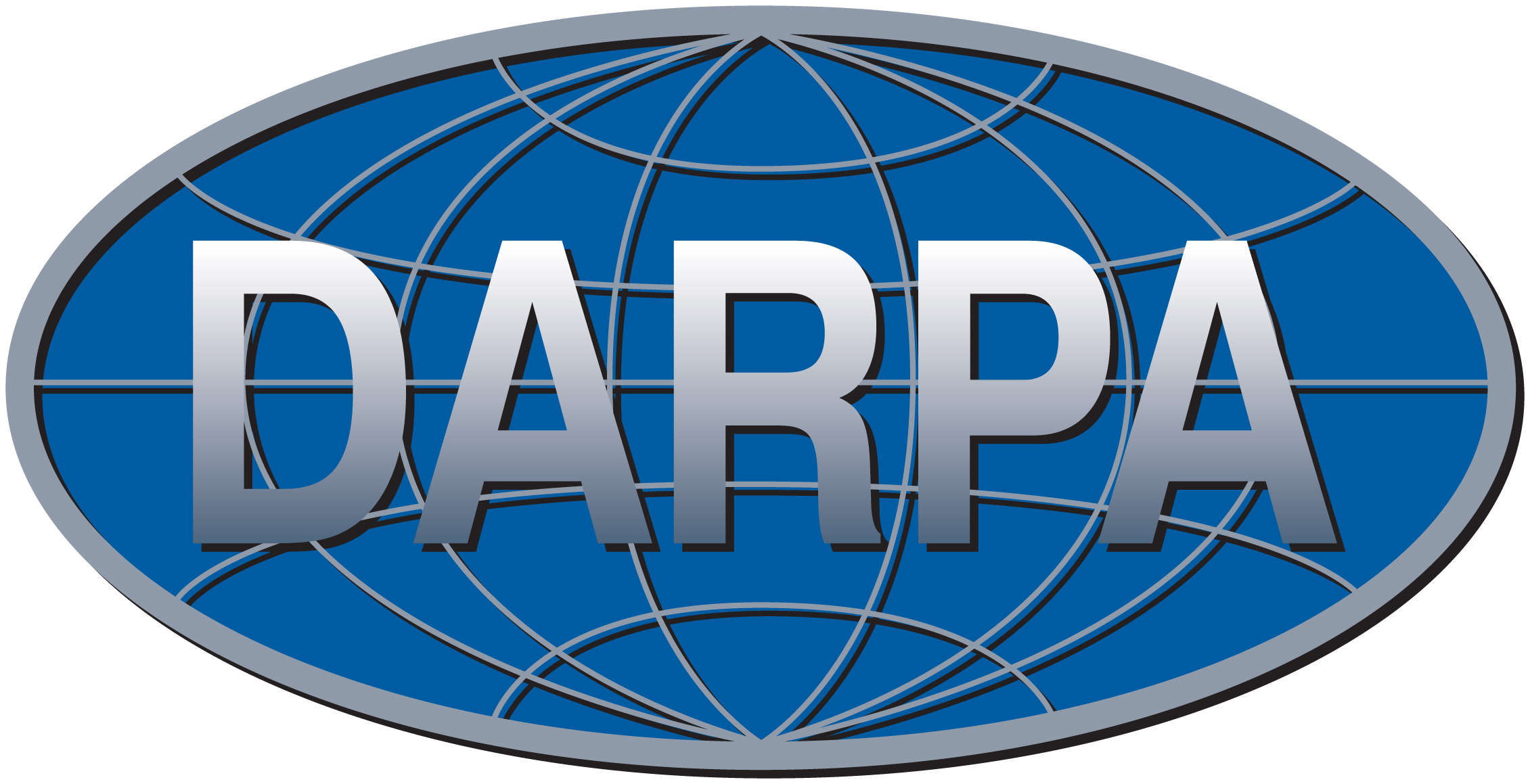CBMS/NSF Conference on Fast Direct Solvers, June 2014

Dartmouth College, June 23 - 27, 2014

 Home Lectures Codes Bibliography Support

Note: The codes provided here are Matlab implementations that were built for educational purposes. They are not in any way optimized, and are not intended for serious computations.
• Randomized SVD (RSVD)

Some tutorial style implementations of the RSVD are provided here. There is GPU support via Matlab (for supported machines).

For a more serious implementation, see the by "ID" package Mark Tygert.

An optimized suite of software written in C is currently being prepared, and will be released in the fall. It will use the MKL libraries, and will include support for GPU computing using CUDA. Once it is complete, it will be announced here.

• Structured matrix algebra

This code contains programs for manipulating "S-matrices" (the "simple" format for rank-structured matrices).

A demonstration code showing how HBS matrix algebra can be used to solve BIEs in 2D can be accessed here (zipped version).

• The Fast Multipole Method (FMM)

A tutorial style Matlab implementation of the FMM can be downloaded here.

For serious implementations of the FMM, check, e.g., here or here.

A general collection of references on the FMM can be found here.

• Interpolative decomposition (ID)

This auxiliary function computes interpolatory decompositions. Note that the necessary functions are not incorporated in Matlab. As a consequence, this code is set up to operate in either one of two modes: Matlab mode ('MAT'): It computes a full SVD of A, and then constructs the ID from this. (If A is large, this is NOT efficient.) Pivoted Gram-Schmidt mode ('PGS'): Pivoted QR with "paranoid" reorthogonalization. This works well but is slow. (To achieve both high efficiency and high accuracy, "mex"-functions must currently be used.) To test "id_decomp.m" use test_id_decomp.m.

The figures in Lecture 3 that compare the errors incurred by the SVD, the ID, multipole expansions, and RSVD were generated by the following files: Laplace, Helmholtz.The codes provided for computing skeletons are all unsatisfactory. What we would need is a matlab function for computing a partial factorization (QR, SVD, LU, ...). This does not currently exist, so we a left with the choice of either using full factorizations (complexity O(N3)), or hard-coding O(N2k) inefficient partial factorization algorithms. The most desirable solution would be to have partial rank factorizations built-in to Matlab. Until we get that, the proper solution is to compile a Fortran or C implementation using the "mex-file" framework. Work on developing these is in progress - please check this website in a few months. A work around is to use the rsvd.

• Direct solvers for sparse matrices

This code sets up a standard rectangular grid. It also computes some stiffness matrices associated with various 5-point stencils. It tests the codes by solving Laplace and Neumann problems, and plots eigenvectors.

Code illustrating the compressibility of "frontal matrices" is available here.

A full implementation of the nested dissection scheme for the classical 5-point stencil is available here (zipped version).

• Boundary integral equations

For illustrations of high order quadrature rules for BIEs, check this website.

The package MPSPACK by Alex Barnett can be explored here.

Let us repeat the link to a demonstration code showing how HBS matrix algebra can be used to solve BIEs in 2D here (zipped version).

A set of tutorial codes on BIEs can be downloaded here (zipped version).

Codes for modeling a simplistic scattering problem are available here.

• Spectral methods

Currently, we unfortunately do not have a public release of the code for the Hierarchical Poincare-Steklov scheme. Hopefully, this will be available within not too long. (If so, it will be posted here.)

In the meantime, let us simply point to this code which sets up spectral discretizations of the Laplace equation on a square or rectangle. It tests the code on Dirichlet problems for Laplace and Helmholtz. Eigenvectors are computed. (Compare the accuracy in the evals to those shown in Lecture 3 for the 5-point stencil!) It sets up a problem on a composite domain consisting of two Cartesian grids.

A wonderful illustration of the utility of high order polynomial approximation is provided by the chebfun package developed at Oxford. For anyone wanting to learn about spectral methods, the codes available at the website for the book "Spectral methods in Matlab" by Lloyd Trefethen are very useful. The function "cheb" which construct Chebyshev nodes and a differentiation matrix is available here.

Research support by:P.G. Martinsson, June 2014